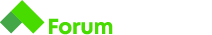# Задача

Здравейте!
Постоянно ми дава грешка и не знам как да я реша тази задача.
Хелп, плс!

# Tips

You are at a restaurant and the waiter brings you the bill. You will have to calculate how much the tip must be. The tips are different in different countries, but here we will use 10%.

## Input

• On the first line you will receive the price of the meal you ordered.

## Output

• You should print the total sum you have to pay (including the tip: 10%)

## Constraints

• The final sum will be always a whole number. You don’t have to print anything after the decimal point.
``````bill = int(input())
print(round(bill * 1.1))
``````

Първо декларираме променлива,която ще съхранява входните данни като целочислен тип.
След това принтираме директно входните данни умножени по 1,1 защото от нас се иска просто да увеличим числото с 10% и закръгляме с вградената “round” функция в Python.

``````bill = 10
percentage = 10 / 100
tip = percentage * 10
result = bill + tip
print(round(result))
``````

Ето по-раздробения вариант с примерна сметка 10.

bill = float(input())

tip = bill * 0.1 + bill ;

print(round(tip));